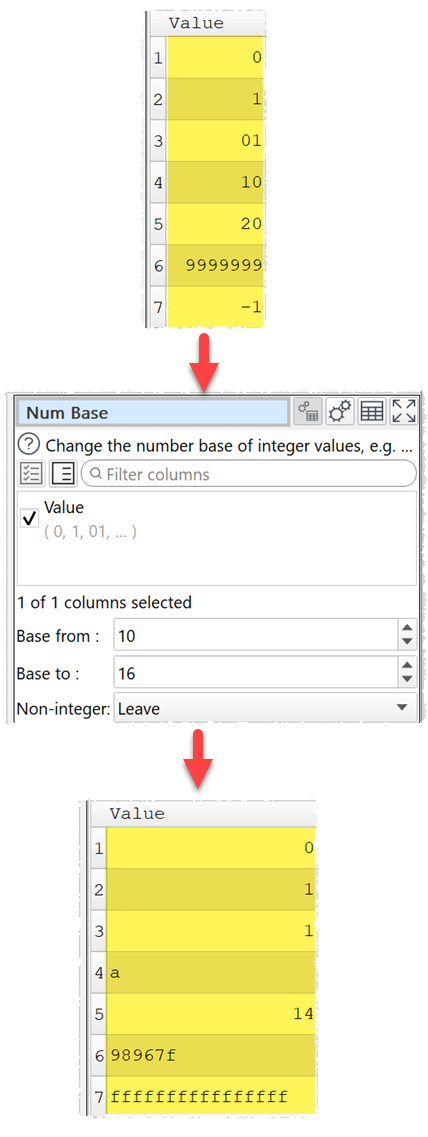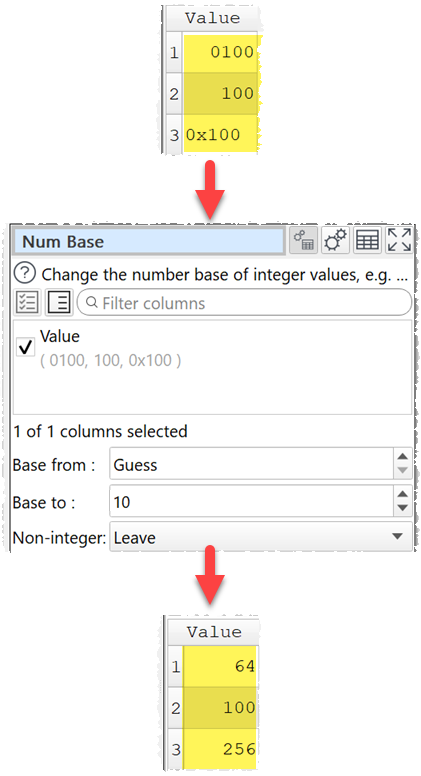﻿ Reference > Transforms > Num Base

# Num Base

## Description

Change the number base of integer values, e.g. decimal to hexadecimal.

## Examples

Convert from decimal (base 10) to hexadecimal (base 16):Convert from guessed base to decimal (base 10):One.

## Options

Check the column(s) you wish to convert.

Set Base from to the base of the current values. If you set it to Guess, it will guess the base for each value using C conventions:

oNumbers starting with 0x (e.g. 0x123) are assumed hexadecimal (base 16).

oNumbers starting with 0 (e.g. 0123) are assumed octal (base 8).

oAll other values (e.g. 123) are decimal (base 10).

Set Base to to the base wanted.

## Notes

The values to be converted should be integers (whole values) without group separators (e.g. no commas or decimal points).

If Base from and Base to are the same, no change is made.

Negative numbers are represented using unsigned values (e.g. twos complement for binary) if Base to is anything other than 10.

Warnings are shown in the Warnings tab for non-integer values.

Use Copy Cols to copy columns before conversion.

Use Num Format to change the format of numbers (e.g. remove group separators).

You can use Insert to add characters to the front of values, e.g. to add '0x' to hexadecimal values.